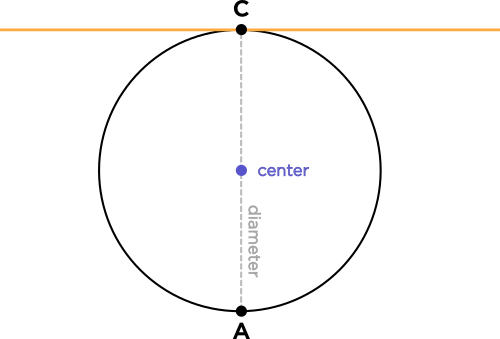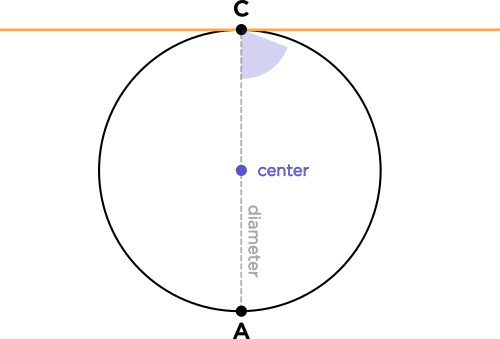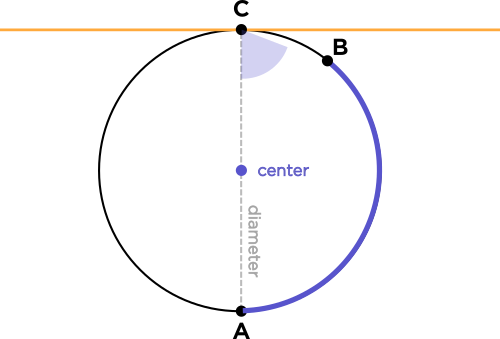# But then can't the other side with 180 degrees make up for the 4 degrees the 176 degree arc missed?

• Module 2 Week 3 Day 9 Challenge Part 2

Then can't the other side with 180 degrees make up for the 4 degrees the 176 degree arc missed or something? I see the logic sort of but I don't get why the tangent would then be intersecting the circle in 2 places.

• @TSS-Graviser The logic can be quite confusing here!

Why does $$A$$ have to be where it is? We're fixing the point $$A$$ at the place where it is because that is the point exactly half of the circle across from the tangent point. This is a really important detail, and it is integral to the whole proof, because we are trying to show that we must get a right angle at $$\overline{AC}$$ and the tangent line. If $$AC$$ doesn't go across half the circle, then it won't go through the circle's center. And since the whole point is to prove that a radius is perpendicular to the tangent line, we really need $$\overline{AC}$$ to go through the center.

We are sort of pretending to be blind in the course of proving this fact! We are pretending that we can only use arc measures and inscribed angles, without eyeballing the angle between $$\overline{AC}$$ and the tangent line. Mathematicians like to do this to themselves, blindfold themselves. It's a process of proving without a doubt that something is true!• Thanks! Also if there is another inscribed angle, how does the tangent now connect in 2 places?

• @TSS-Graviser The first intersection point is point $$C$$, which we get before we mess with the nice pure diagram of a circle with just a tangent line:Now we're going to fix point $$A$$ to be the other endpoint of the diameter which passes through $$C$$, so that we get the nice property that $$\overline{AC}$$ contains a radius.This is a proof by contradiction.We want to prove that the angle between $$\overline{AC}$$ and the tangent line is $$\angle 90^{\circ}.$$ So, let's suppose that it's not $$\angle 90^{\circ}$$ and try to get something ridiculous!

Now it's time to pretend to be blind, because we're going to imagine that this angle is not a right angle, so it looks like the purple angle in this picture:(Which clearly looks wrong to our eyes, but bear with us!) The purple angle inscribes less than a $$180^{\circ}$$ arc of the circle, which we can color purple here:This tells us the odd fact that the point $$B,$$ where the arc ends, must be on the purple angle's lines, which means that the yellow line (which is supposed to contain the purple angle), has a point at $$B,$$ which means it intersects the circle twice..... which is wrong!

$$\implies$$ The purple angle must actually be a right angle, and the yellow tangent line is perpendicular to the circle's radius.

Don't worry if your head seems to feel funny, because this is a proof by contradiction, so things shouldn't be making sense!• Where is the point at B? Is there a visual?

• Sorry if I'm getting annoying.

• @TSS-Graviser The point at $$B$$ is just the endpoint of the purple arc, which can be of an arbitrary length, as long as it is less than a semicircle!

• I mean how does the tangent intersect B? As far as I can see it's still going straight and shouldn't be touching it.

• @TSS-Graviser You're not being annoying; you're actually seeding new content for everyone else to benefit from. These explanations get saved, so that everyone else wondering about the same questions can see them.• @TSS-Graviser Yes, it's because the construction of a tangent not perpendicular to the radius is inherently possible, which is why the diagram just looks wrong.But when you prove things, you can't just say, "It looks like it..." You instead refer to theorems, facts or properties that are unambiguous. For now it's just very weird, but being able to prove things, while onerous at times, makes it nice for us, because we can put total faith in our assumptions and calculations.